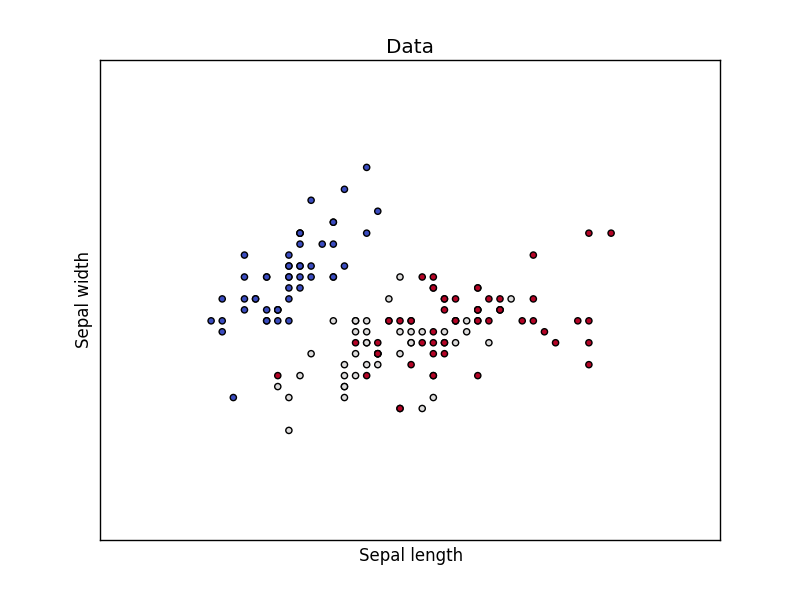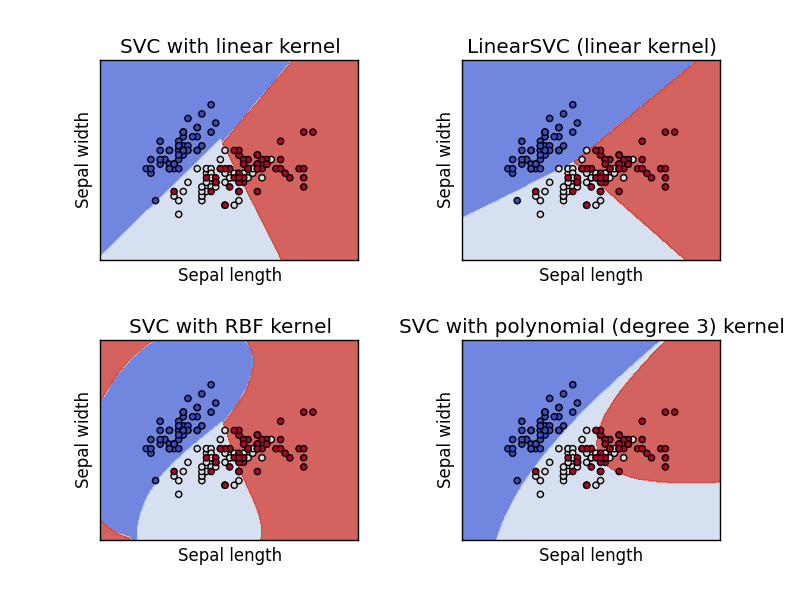# Support Vector Machine

Python hosting: Host, run, and code Python in the cloud!

A common task in Machine Learning is to classify data. Given a data point cloud, sometimes linear classification is impossible. In those cases we can use a Support Vector Machine instead, but an SVM can also work with linear separation.

Related Course:

Dataset
We loading the Iris data, which we’ll later use to classify. This set has many features, but we’ll use only the first two features:

• sepal length
• sepal width
The code below will load the data points on the decision surface.Support Vector Machine Example
Separating two point clouds is easy with a linear line, but what if they cannot be separated by a linear line?

In that case we can use a kernel, a kernel is a function that a domain-expert provides to a machine learning algorithm (a kernel is not limited to an svm).

The example below shows SVM decision surface using 4 different kernels, of which two are linear kernels.